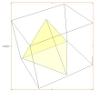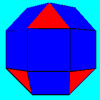#### You may also like### A Mean Tetrahedron

Can you number the vertices, edges and faces of a tetrahedron so that the number on each edge is the mean of the numbers on the adjacent vertices and the mean of the numbers on the adjacent faces?### Rhombicubocts

Each of these solids is made up with 3 squares and a triangle around each vertex. Each has a total of 18 square faces and 8 faces that are equilateral triangles. How many faces, edges and vertices does each solid have?### Icosian Game

This problem is about investigating whether it is possible to start at one vertex of a platonic solid and visit every other vertex once only returning to the vertex you started at.

# Which Solids Can We Make?

### Why do this problem

This problem builds on work done in finding Semi-Regular Tessellations and offers a great opportunity to create the Archimedean solids using students' knowledge of interior angles of polygons and angles around a point.

### Possible approach

These printable worksheets may be useful: Which Solids Can We Make?
Which Solids Can We Make? - Extension

The problem has been structured in such a way that a class can spend some time answering each of the questions in bold, with time set aside to discuss approaches, thoughts and solutions.

The problem builds up to the search for Archimedean solids using what students learn about the angle deficit of Platonic solids. One way of introducing the concept of angle deficit could be to make a solid out of Polydron and then unfold it into its net and look at the gap at a vertex.

You might also want to make a link between exterior angles in two dimensions and angle deficit in three dimensions:

"In two dimensions, the interior angles of convex polygons are always less than $180^{\circ}$. Can you explain why?"
"In three dimensions, the sum of the interior angles at each vertex of convex polyhedra must be less than $360^{\circ}$. Can you explain why?"

"The exterior angles of polygons are a measure of how far short the angles are from $180^{\circ}$."
"The angle deficit at a vertex of a polyhedron is a measure of how far short each angle sum is from $360^{\circ}$."

Once students are happy with the concept of angle deficit, you could organise their results into a table like this:

 Vertex Form Angle Sum Angle Deficit Number of Vertices Total Angle Deficit Cube $4, 4, 4$ $270$ $90$ $8$ $720$ Tetrahedron Octahedron Icosahedron Dodecahedron

This leads nicely to the observation that the total angle deficit is constant.

For teachers with access to Polydron or similar construction sets, the last part of the problem provides a fruitful opportunity for much rich mathematical thinking, maybe spanning more than one lesson.

One way of scaffolding the search for more Archimedean solids is to list factor pairs of 720, and then challenge students to find potential angle deficits which are factors of 720. This could be done in small groups, using Polydron to check any possibilities they come up with.

NB Not all angle deficits which are factors of 720 can be made into solids - this is the three dimensional analogy of patterns such as {10, 5, 5} in the problem Semi-Regular Tessellations.

A list of all the Archimedean solids, their vertex forms and their names can be found in this article.
NB This list would be infinitely long if we included prisms and antiprisms so this is why they are excluded from the definition of Archimedean solids.

### Key questions

Why do the interior angles meeting at a vertex always add up to less than $360^{\circ}$?
If you had a small number of vertices, would you expect the angle deficit to be large or small?
(Hint: think about how "pointy" a tetrahedron is compared with a dodecahedron)

### Possible support

Starting with some two-dimensional work on Semi-Regular Tessellations may help to prepare students for the three-dimensional thinking required in this task.

Explore the total angle deficit of prisms and antiprisms before moving onto more complicated solids.

### Possible extension

Once students have suggested a possible vertex form, challenge them to explain how they could work out how many of each type of polygon will be required to make the solid.